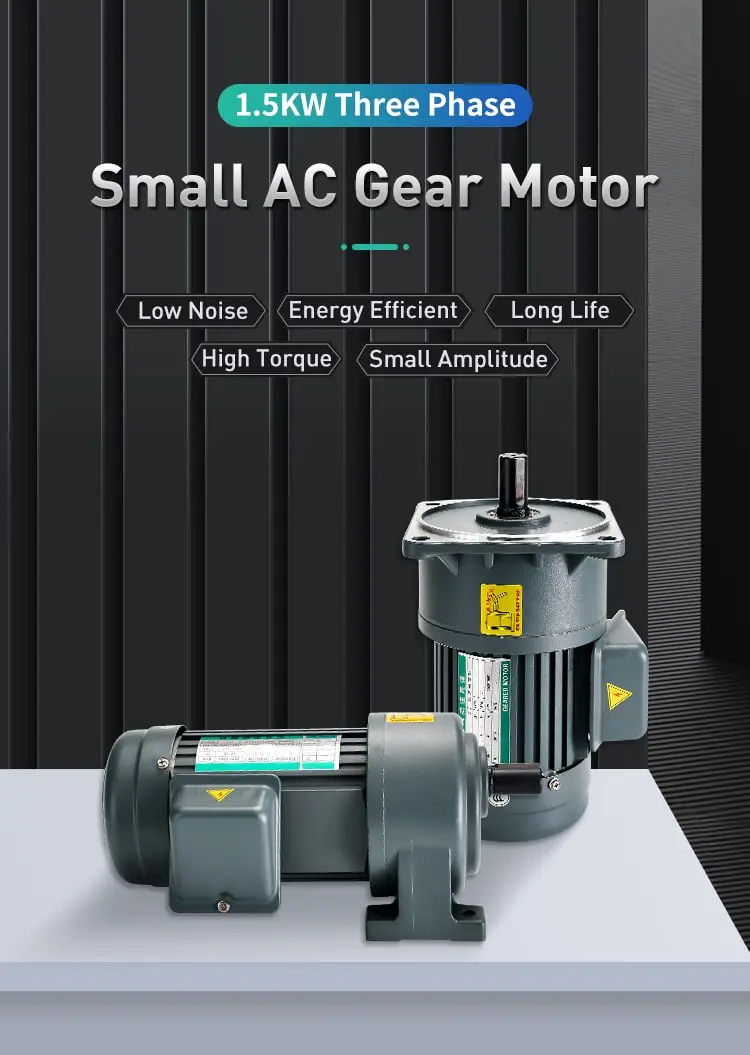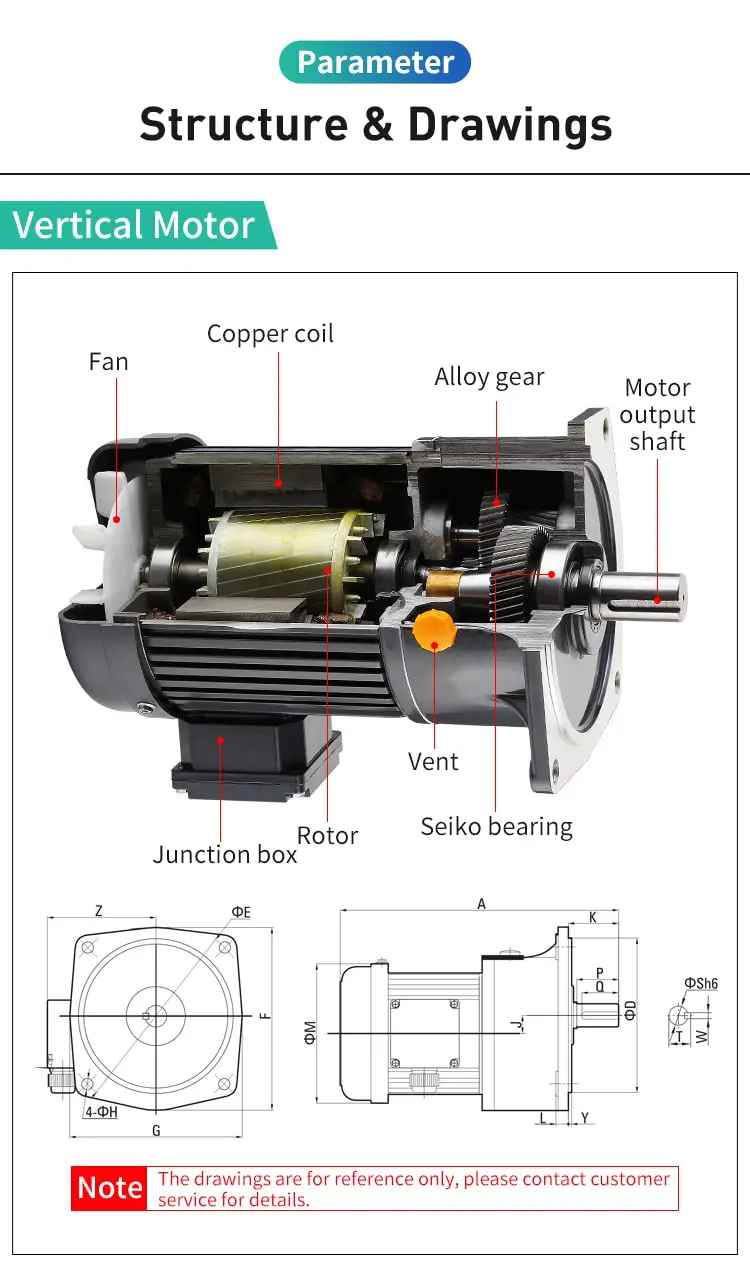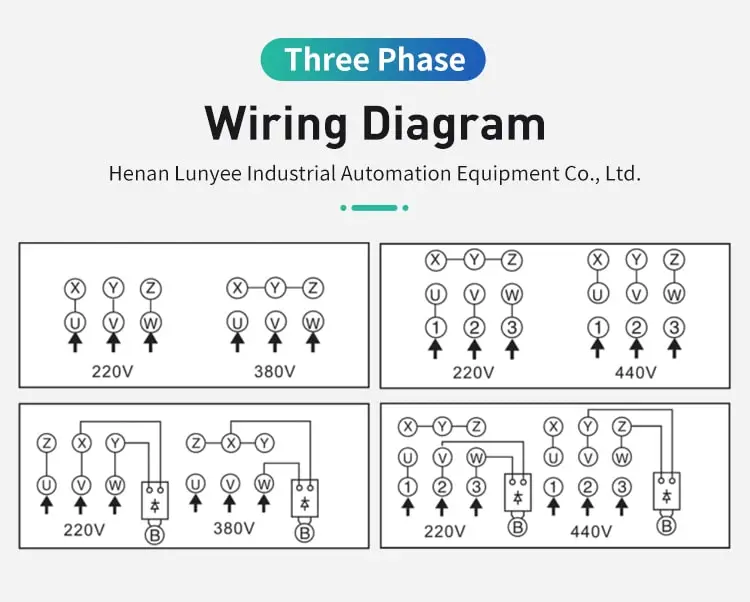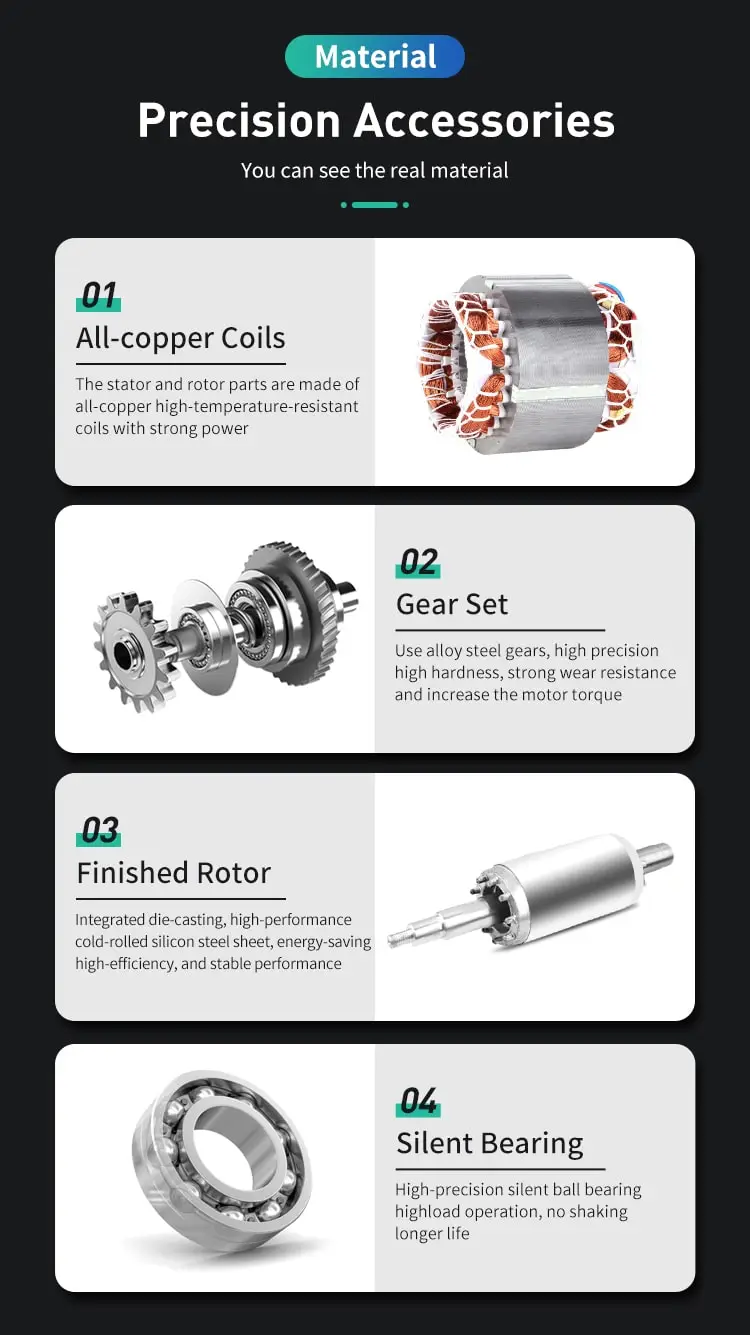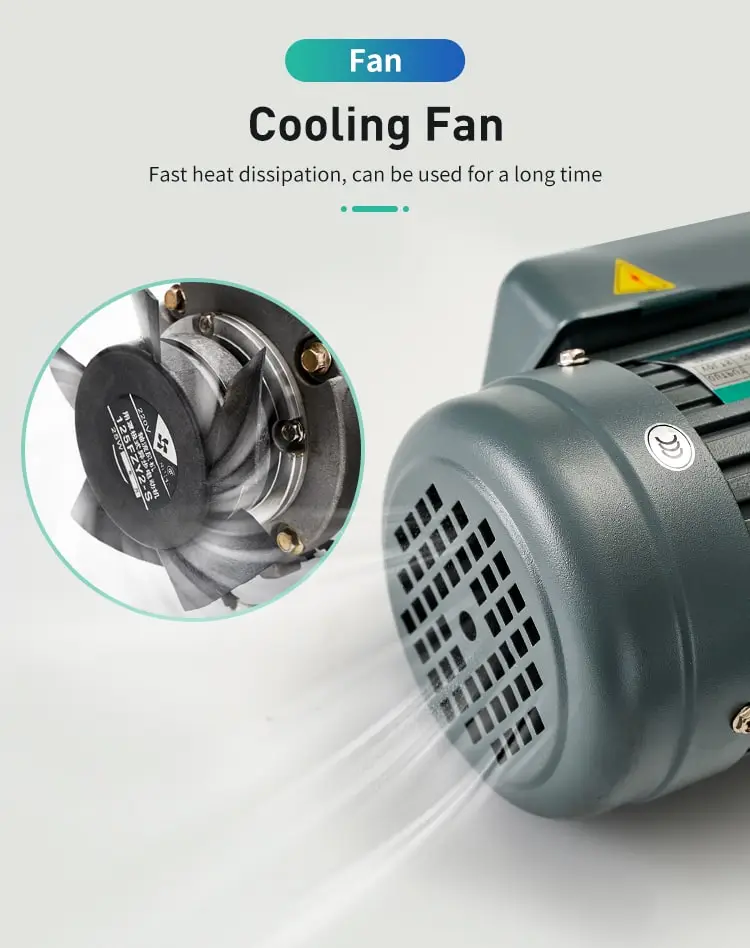By Jasmine 2023-11-07 16:51:25

#### How to make a servo motor turn 90 degrees?

Servo motors enable precise movements in robotics. To achieve a 90-degree rotation, understand servo basics, calculate pulse width, and control the motor with PWM signals.

In the realm of robotics and automation, servo motors play a crucial role in enabling precise and controlled movements. These motors, unlike conventional DC motors, can accurately position their shaft to a specific angle, making them ideal for applications like robotic arms, animatronics, and model airplanes. Among the common tasks involving servo motors is rotating them to a desired angle, such as 90 degrees.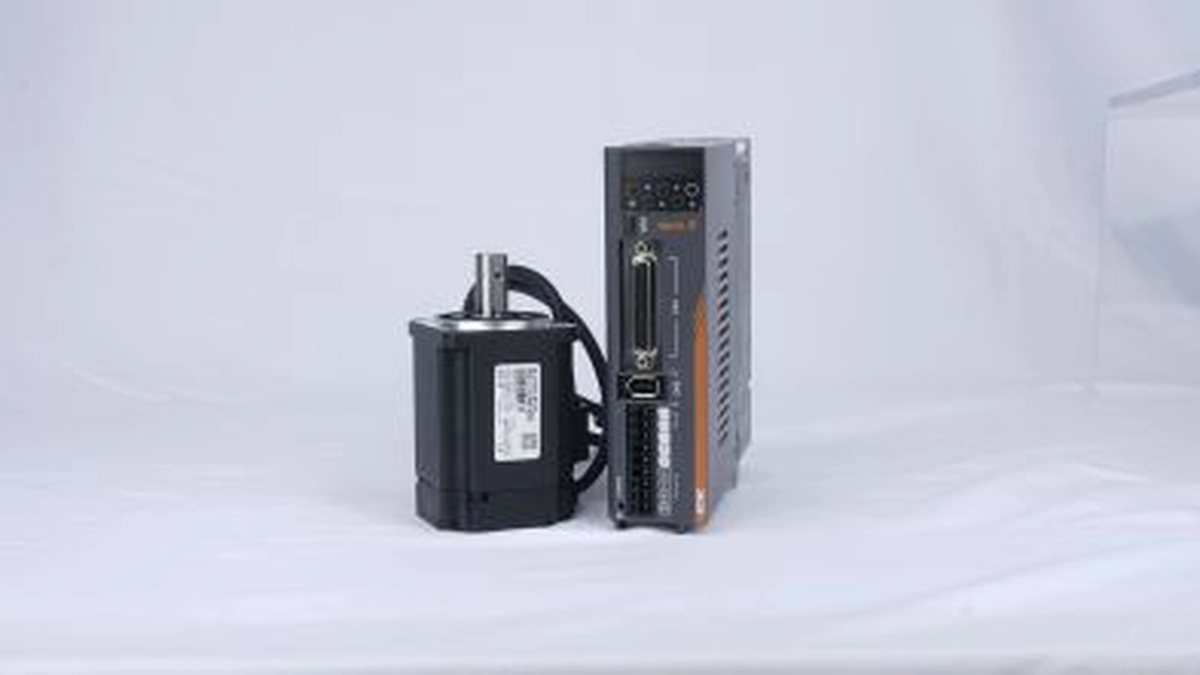## Understanding Servo Motor Basics

To effectively control a servo motor and achieve a 90-degree rotation, it's essential to grasp the fundamental concepts of servo operation. Servo motors receive control signals in the form of pulse width modulation (PWM) signals, which are essentially square-wave pulses with varying pulse widths. The pulse width within a specified range determines the position of the servo motor's shaft.

## Calculating the Pulse Width for 90-degree Rotation

The pulse width range for a typical servo motor lies between 1 and 2 milliseconds (ms). A pulse width of 1 ms corresponds to the servo motor's minimum angle, while a pulse width of 2 ms corresponds to its maximum angle. To achieve a 90-degree rotation, the pulse width needs to be calculated based on the servo motor's specific pulse width range and the desired angle.

## Controlling the Servo Motor with PWM Signals

Several methods can be employed to generate and transmit PWM signals to the servo motor. One common approach involves using a microcontroller, such as an Arduino, programmed to generate the appropriate PWM signals based on the desired angle. The microcontroller's output pins can be connected to the servo motor's control pin, enabling precise control of its position.

## Implementing the 90-degree Rotation

With the pulse width calculated and the PWM control method chosen, the 90-degree rotation can be implemented. Here's a step-by-step guide:

1. Connect the servo motor:< Connect the servo motor's power supply, ground, and control pins to the corresponding power source and controller (microcontroller or other PWM generator).

2. Calculate the pulse width:< Determine the pulse width corresponding to 90 degrees using the servo motor's pulse width range.

3. Generate the PWM signal:< Generate the PWM signal with the calculated pulse width using the chosen control method (microcontroller, PWM generator, etc.).

4. Apply the PWM signal:< Apply the generated PWM signal to the servo motor's control pin.

## Conclusion

Rotating a servo motor to a specific angle, such as 90 degrees, requires understanding the principles of servo operation, calculating the appropriate pulse width, and implementing PWM control. By following these steps, you can effectively control servo motors and achieve precise positioning in various applications.